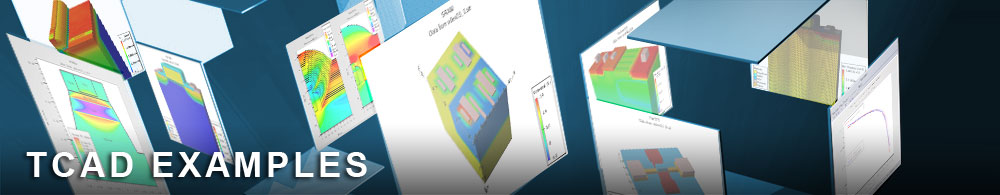Dynamic Simulation of Relaxation Oscillation

## laserex06.in : Dynamic Simulation of Relaxation Oscillation

Requires: Blaze/Laser
Minimum Versions: Atlas 5.28.1.R

This example demonstrates the simulation of the buried heterostructure InP/InGaAsP laser diode described earlier in example laserex02. A description of the structure and the DC behaviour is contained in example laserex02. This example performs a transient simulation of an impulse applied to the anode contact of the laser diode.

The key points of this simulation are :

• Mulit-longitudinal mode simulation
• Addition of laser losses of bulk absorption and free carrier loss
• DC simulation to obtain the operating point at the lasing threshold
• Transient simulation response to a small impulse at the anode contact
• Resultant oscillation in simulated photon density

The dynamic laser equations used in Atlas include the photon rate equation as well as the equations for gain and spontaneous recombination. This set of equations will be shown in this example to reproduce the dynamic characteristics of relaxation oscillation.

The example described in laserex02 for DC characteristics of a laser diode has been extended to examine the relaxation oscillations that exist when a sudden impulse is applied to the anode contact. This requires a transient simulation of the laser to reproduce these oscillations. The physical origin of the oscillation is is based upon the following principle: an increase in carrier density in the laser (caused by the small impulse) will cause an increase in the calculated gain which results in a rise in the photon density inside the laser. This increase in photon density causes an increase in the stimulated recombination rate which decreases the carrier density, which causes the photon density to fall, which causes the carrier density to rise, and so on. An oscillatory process is therefore established which will have some characteristic frequency and a characteristic damping time constant.

This simulation uses the multi-longitudinal mode calculation, first used in laserex02, in both the DC and transient parts of the simulation. The final plots will illustrate how the laser emission spectrum will change from the beginning to the end of the transient simulation.

Four laser loss mechanisms have also been included; internal losses, mirror loss, bulk absorption loss and free carrier loss. These mechanisms have been activated with the parameters las.losses, las.mirror, las.absorption and las.fcarrier.

The plot of the final logfile produced by the transient simulation will plot the photon density versus time, and will show the oscillation described previously.

To load and run this example, select the Load button in DeckBuild > Examples. This will copy the input file and any support files to your current working directory. Select the Run button in DeckBuild to execute the example.